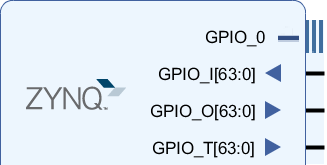# PS GPIO¶

The Zynq device has up to 64 GPIO from PS to PL. These can be used for simple control type operations. For example, in the base overlay, the PS GPIO wires are used as the reset signals for the IOPs. The PS GPIO are a very simple interface and there is no IP required in the PL to use them.

The GPIO class is used to control the PS GPIO. Note that AXI GPIO are controlled by the AxiGPIO class.

## Block Diagram¶## Linux GPIO¶

The PS GPIO use a Linux kernel module to control the GPIO. This means that the operating systems assign a number to the GPIO at run time. Before using the PS GPIO, the Linux pin number must be mapped to the Python GPIO instance.

The get_gpio_pin() function which is part of the GPIO class is used to map the PS pin number to the Linux pin number. See the example below on how it can be used.

## Example¶

The PS GPIO need to be connected to something in an overlay before they can be used. The example below is for illustration. It shows a code snippet that needs an appropriate overlay with the PS GPIO connected to something.

from pynq import GPIO

output = GPIO(GPIO.get_gpio_pin(0), 'out')
input = GPIO(GPIO.get_gpio_pin(1), 'in')

output.write(0)